Open in App
Not now

# Grouping of Data – Definition, Frequency Distribution, Histograms

• Last Updated : 11 Nov, 2021

Data Handling or Handling of data is not just a mathematical term but is used in everyday life. When there is a requirement of recording, gathering, and presenting any type of information or data, data handling is preferably used. Statistics is a word we often hear, is not but another term for data handling. From making a bar chart of favorite candies of different students to representing a large survey done on the Covid-19 cases, data handling is used and preferred.

A lot of times we come across information such as:

1. Number of Covid Cases in past few months.
2. Number of Goals scored by a team in World Cup

This information in such cases is called Data. Data can be represented in both statistical ways and graphical ways. Graphical ways usually visually appealing are also easier to understand by a common person. There are many ways to represent data graphically:

1. Pictograph
2. Bar Graph
3. Double Bar Graph

Now the question comes how to handle data and prepare it in such a way that it can be represented by these graphs.

### Introduction to Data Handling

Data Handling is the process of gathering, recording, or presenting information in such a way that is helpful to others in instances like graphs or charts.

Usually, the data that we receive is not organized. This kind of data is called raw data. To present it in a meaningful way or to draw good conclusions out of it we need to organize it systematically. For example, consider the following data,

Students of Literature were asked to name their favorite existentialist author. The results are listed below:

Camus, Kafka, Nietzsche, Camus, Camus, Nietzsche, Kafka, Camus, Camus, Kafka, Kafka, Kafka, Kafka, Camus, Camus, Nietzsche, Kafka, Camus, Kafka, Kafka

Now the question we need to answer is which was the least liked author?

It’s not easy to count like this if this data had been huge. It would have been impossible to count like this. That’s why we need to organize the data.

### Data Grouping

The previous example can be solved by grouping data properly. It can be easier to count if these values were grouped with their type. Let’s do that for the previous example only,

We have the following data:

Camus, Kafka, Nietzsche, Camus, Camus, Nietzsche, Kafka, Camus, Camus, Kafka, Kafka, Kafka, Kafka, Camus, Camus, Nietzsche, Kafka, Camus, Kafka, Kafka

We see that there are three entities here: Camus, Kafka, and Nietzsche. Let’s count their occurrences and group them by their occurrences in a table.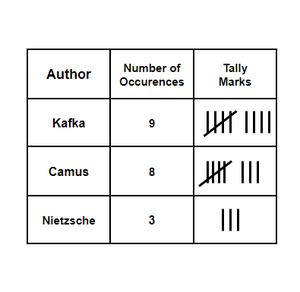The lines and crosses on them are called tally marks. They sometimes make it easier for us to count the number of objects. Now we can see that the least liked author is Nietzsche. The value in the “Number of Occurrences” column is called the frequency of that entity and this table is called frequency distribution.

### Grouped Frequency Distribution

Sometimes it can happen that, there too many values in a particular range. So, it can be really difficult to make a frequency table for each entity. Instead, we make a frequency table for a range and count the number of entities lying in that range.

For example,

Let’s say we have data that shows the runs made by a particular batsman in 60 matches.

21, 10, 30, 22, 33, 5, 37, 12, 25, 42, 15, 39, 26, 32, 26 27, 28, 19, 29, 35, 31, 24, 36, 18, 20, 38, 22, 44, 16, 24, 10, 27, 39, 28, 49, 29, 32, 23, 31, 21, 34, 22, 23, 36, 24, 36, 33, 47, 48, 50, 39, 20, 7, 16, 36, 45, 47, 30, 22, 17

Now we cannot make a frequency table for each value, thus we use the grouped frequency distribution concept discussed above.

Let’s make a range like 0-10, 10-20, 20-30, and so on.

Note: Notice that in the interval 0-10, 10-20. 10 is common, but a value cannot belong to two intervals simultaneously. Thus, a convention is assumed that the common observation will belong to higher class. So 10 will belong to the interval 10-20.

In a group 50-60, 50 is called the lower class limit and 60 is called the upper-class limit. The difference between the upper-class limit and the lower class limit is called the width or size of the interval.

### Histograms

It is similar to a bar graph but a histogram groups the numbers into ranges and then plot the occurrences of values in the given ranges on the graph.

Let’s consider the previous example,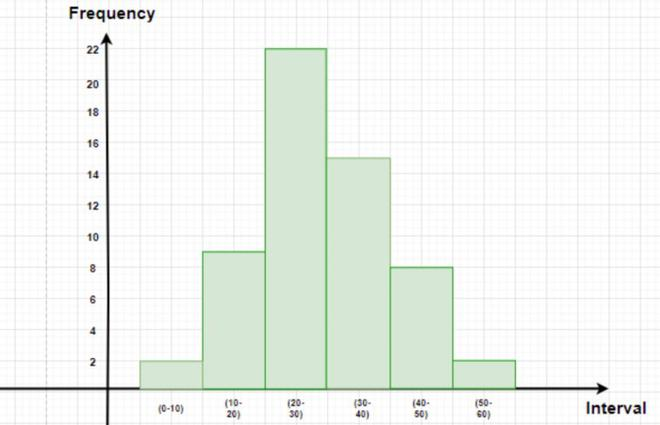The figure below represents this data in graphical format. The height of the bars represents the frequency of the class-interval. Notice that there is no gap between the bars. This kind of graph is called a histogram.

### Sample Problems

Question 1: A die was thrown 25 times and the following scores were obtained:

2, 5, 2, 4, 3, 6, 1, 4, 2, 5, 1, 6, 2, 6, 3, 5, 4, 1, 3, 2, 3, 6, 1, 5, 2

Create a frequency table of the scores.

Solution:

The frequency table of the scores obtained when a die is thrown can be shown as –

Question 2: Make the bar graph for the data given in the literature example in the beginning.

Solution:

Let’s make the table for the given data

Let’s put the names of authors on the x-axis and number of occurrences on the y-axis.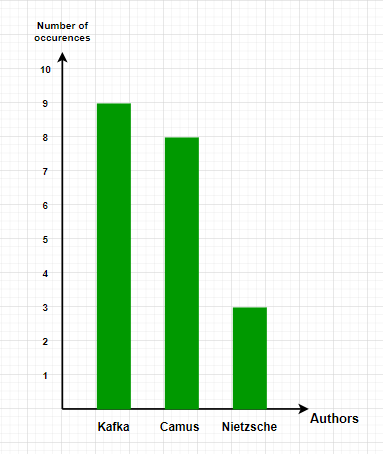Question 3: Make histogram for the data given below:

1, 2, 2, 1, 5, 5, 4, 10, 4, 5, 7, 10, 9, 8, 9, 9, 11

Solution:

Let’s assume an interval size of 3 and make a frequency table.

Let’s plot these intervals on graph.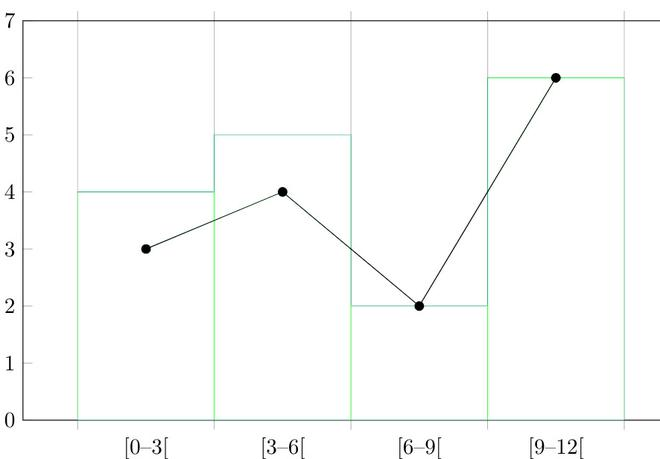Question 4: The data given below represents the usage per day in minutes for Spotify for a particular person.

5, 10, 12, 7, 20, 13, 30, 25, 20, 50, 30, 24, 17, 63, 24, 30, 15, 10, 40, 24, 15, 18, 20, 11.

Make a frequency table and a histogram for this data.

Solution:

Before making a histogram, we need to group the data and make a frequency distribution for it.

Let’s assume the interval size to be 10.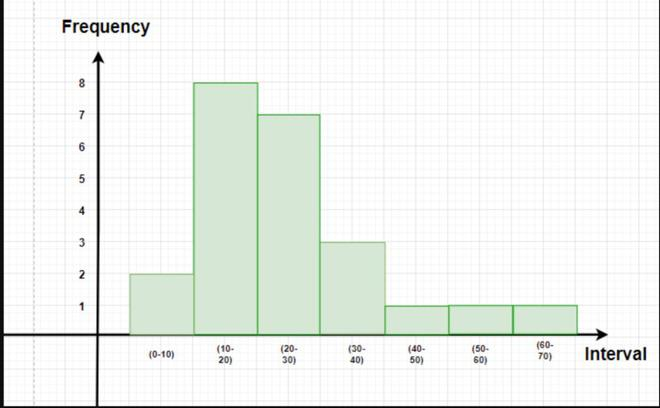Question 5: Answer the following questions by observing the given histogram.

1. What is the information given by the histogram
2. Which group contains the maximum girls.
3. How many girls have marks more than 145.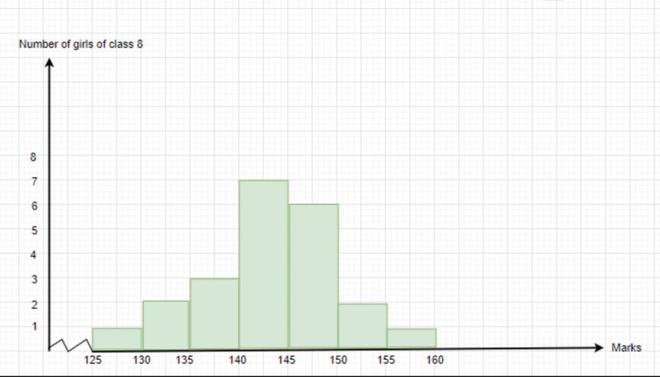Solution:

1. The histogram represents the total marks obtained the girls in the class.
2. The group 140-150 contains the maximum number of girls.
3. 9 girls have marks more than 145.

My Personal Notes arrow_drop_up
Related Articles